Symmetry

(redirected from axisymmetric)
Also found in: Dictionary, Thesaurus, Medical.
Related to axisymmetric: axisymmetric flow

symmetry,

generally speaking, a balance or correspondence between various parts of an object; the term symmetry is used both in the arts and in the sciences. In art and design, it is often used in a somewhat loose sense, to mean a kind of balance in which the corresponding parts are not necessarily alike but only similar. A symmetrical design should produce a pleasing effect; if there is too close a correspondence, the effect may be monotonous. Ancient Greek architecture is particularly distinguished for its symmetry. In modern art, the Dutch artist M. C. Escher achieved a number of striking effects in his works exploring mathematical symmetry. A mathematical operation, or transformation, that results in the same figure as the original figure (or its mirror image) is called a symmetry operation. Such operations include reflection, rotation, double reflection, and translation. The set of all operations on a given figure that leave the figure unchanged constitutes the symmetry groupgroup,
in mathematics, system consisting of a set of elements and a binary operation a+b defined for combining two elements such that the following requirements are satisfied: (1) The set is closed under the operation; i.e.
for that figure. The symmetry groups of three-dimensional figures are of special interest because of their application in fields such as crystallography (see crystalcrystal,
a solid body bounded by natural plane faces that are the external expression of a regular internal arrangement of constituent atoms, molecules, or ions. The formation of a crystal by a substance passing from a gas or liquid to a solid state, or going out of solution (by
). In general, a symmetry operation on a figure is defined with respect to a given point (center of symmetry), line (axis of symmetry), or plane (plane of symmetry). In biology, symmetry is studied in the correspondences between different parts of a given organism, as between the left and right halves of the human body or between the various segments of a starfish (see symmetry, biologicalsymmetry, biological,
similarity or balance between parts of an organism so that when a straight cut is made through a point or along a line, equal, mirror-image halves are formed. Symmetry in body shapes is related to the lifestyles of organisms.
). In physics, basic symmetries in nature underlie the various conservation lawsconservation laws,
in physics, basic laws that together determine which processes can or cannot occur in nature; each law maintains that the total value of the quantity governed by that law, e.g., mass or energy, remains unchanged during physical processes.
. For example, the symmetry of space and time with respect to translation and rotation means that a given experiment should yield the same results regardless of where it is performed, what direction the equipment is pointing in, or when it is performed. These three symmetries can be shown to imply the laws of conservation of linear momentum, angular momentum, and energy, respectively.

Bibliography

See G. E. Martin, Transformation Geometry (1987); B. Bunch, Reality's Mirror (1989); M. C. Escher, Escher on Escher (tr. 1989).

Symmetry

The exact correspondence of forms of similar size and arrangement of parts, equidistant and on opposite sides of a dividing line or plane about the center line or axis.bilateral symmetry

A balanced arrangement of identical similar elements about a central axis.Balanced elements that radiate from a central point.The following article is from The Great Soviet Encyclopedia (1979). It might be outdated or ideologically biased.

Symmetry

(in biology). The phenomenon of symmetry in nature was studied as early as the fifth century B.C. in ancient Greece by the Pythagoreans, who studied it in relation to the development of the doctrine of harmony. In the 19th century symmetry was studied in plants by the French scientists A. P. De Candolle and A. Bravais, in animals by the German scientist E. Haeckel, and in biogenic molecules by the French scientists A. Béchamp, L. Pasteur, and others. In the 20th century biological objects have been studied from the standpoint of the general theory of symmetry by the Soviet scientists Iu. V. Vul’f, V. N. Beklemishev, and B. K. Vainshtein, by the Dutch physical chemist F. M. Jager, and by a group of English crystallogra-phers headed by J. Bernal. Biological objects have also been studied on the basis of dextrorotation and levorotation by the Soviet scientists V. I. Vernadskii, V. V. Alpatov, and G. F. Gauze and by the German scientist W. Ludwig. This research led to the establishment in 1961 of a new specialization known as biosymmetry.

The structural symmetry of biological objects has been studied the most extensively. Research on the symmetry of molecular and supramolecular biological structures from the standpoint of structural symmetry makes it possible to detect in advance the types of symmetry that are possible for the objects and, consequently, the number and types of modifications that are possible. An accurate description can also be made of the external shape and internal structure of any three-dimensional biological object. As a result, concepts of structural symmetry have been extensively used in zoology, botany, and molecular biology.

Structural symmetry is manifested primarily in the form of some kind of regular repetition. In the classical theory of structural symmetry developed by E. S. Fedorov, the German scientist J. F. Hessel, and others, an object’s symmetrical type can be described by the aggregate of its symmetrical elements, or the geometric elements (points, lines, planes) in relation to which similar parts of the object are ordered. For example, in the type of symmetry exhibited by a phlox, a fivefold axis of symmetry passes through the center of the flower: after a rotation of 72°, 144°, 216°, 288°, or 360° about the axis, the flower has the same appearance as it did in the beginning. A butterfly’s symmetry is characterized by a single plane that divides the butterfly into left and right halves. The symmetry operation involved in this case is reflection, which maps the left half of the figure of the butterfly onto the right half and the right half onto the left half in such a way that, after the reflection, the figure is the same as it was in the beginning. The type of symmetry exhibited by the ra-diolarian Lithocubus geometricus is characterized by a center of symmetry in addition to axes of rotation and planes of reflection. Any straight line drawn within the radiolarian through such a unique point meets “identical” (corresponding) points at equal distances on both sides of the figure. The symmetry operation here is reflection in a point, after which the figure of the radiolarian has the same appearance as it did in the beginning.

Because of various limitations, significantly fewer types of symmetry are usually found in nature than are theoretically possible. For example, plants and animals of the lower stages of development are characterized by representatives of all classes of punctuate symmetry, including organisms having the symmetry of spheres and regular polyhedrons. Plants and animals of the higher levels of evolution are characterized by symmetry that is principally axial (type ri) or actinomorphic (type nm (in both cases n may have a value from 1 to 00). Biological objects with axial symmetry are characterized only by an axis of symmetry of order n. Biological objects with actinomorphic symmetry are characterized by a single axis of order n and by planes m that intersect each other along the axis. In nature, the most common symmetry is of the type n = 1 (asymmetry) and 1 m = m (bilateral symmetry). Asymmetry is characteristic of the leaves of the majority of plant species, and bilateral symmetry is characteristic to a certain degree of the external form of the body of vertebrates, including man, and many invertebrates. In mobile organisms bilateral symmetry is apparently associated with differences in up-down and forward-backward movements, at the same time that left-right movements are the same.

The disruption of bilateral symmetry inevitably leads to the inhibition of the movement of one side and to the transformation of forward motion into circular motion.

Dissymmetric biological objects have been intensively studied since the 1950’s, primarily in the USSR. They may exist in at least two modifications—in the form of an original and the original’s mirror image (antipode). One of these forms (either one) is called the right, or D-form (from the Latin dextro), and the other is called the left, or L-form (from the Latin levo). In studying the forms and structures of D- and L-biological objects, a theory of dissymmetrizing factors was developed, which demonstrated the possibility of two or more modifications for any D- or L-object and simultaneously contained formulas for determining the number and types of modifications. This theory led to the discovery of biological isomerism, or the relation of different biological objects having the same composition.

In studying biological objects, it was established that in some cases D-forms predominate, and in others, L-forms; in some cases, D- and L-forms are equally represented. In the 1840’s, Béchamp and Pasteur and in the 1930’s the Soviet scientist Gauze and others demonstrated that the cells of organisms are constructed only or predominantly of L-amino acids, L-proteins, D-deoxyribonucleic acids, D-sugars, L-alkaloids, and D- and L-terpenes. This very fundamental and characteristic feature of living cells, which Pasteur called protoplasmic dissymmetry, ensures the cell (as has been established in the 20th century) of a more active metabolism. Protoplasmic dissymetry is maintained through complex biological and physicochemical mechanisms, which have arisen in the process of evolution. In research conducted in 1952 on 204 species of vascular plants, Alpatov established that 93.2 percent of the species were of the type with an L-form of helical thickenings of the vascular walls, 1.5 percent were of the type with a D-form, and 5.3 percent were of the racemic type, with the number of D-vessels approximately equal to the number of L-vessels.

In studying D- and L-biological objects it has been established that equality between D- and L-forms in many instances is disrupted because of differences in their physiological, biochemical, and other properties. A similar characteristic in nature has been called the dissymmetry of life. Thus, the stimulating influence of L-amino acids on the movement of plasma in plant cells is tens and hundreds of times greater than the effect of their D-forms. The D-forms of many antibiotics, including penicillin and gramicidin, are more bactericidal than the forms containing L-amino acids. The more commonly found spiral L-roots of the sugar beet are 8–14 percent (depending on the variety) heavier and contain 0.5–1 percent more sugar than the D-roots.

The study of the inheritance of characteristics in D- and L-forms has shown that their dextrorotation or levorotation may be hereditary or nonhereditary or may have the character of prolonged modification. This signifies that, in at least a number of cases, the dextrotation and levorotation of organisms or their parts may be changed by the action of mutagenic or non-mutagenic chemical compounds. Specifically, D-strains (according to the morphology of the colonies) of the microorganism Bacillus mycoides grown on agar with D-sucrose, L-digitonin, and D-tartaric acid can be converted into L-strains, and L-strains grown on agar with L-tartaric acid and D-amino acids can be converted into D-strains.

In nature, the interconversion of D- and L-forms may also occur without human intervention. Changes in the type of symmetry have also occurred during evolution in organisms that are not dissymmetric, and as a result, numerous evolutionary types of symmetry have arisen that are specific for particular branches of the tree of life.

The structural symmetry of biological systems is also studied from the standpoint of more general types of symmetry, for example, color symmetry, the symmetry of similitude, and antisymmetry.

Further studies on the symmetry of biological objects will increase our knowledge about the properties and functions of the objects, as well as about the origin and essence of life.

REFERENCES

Gauze, G. F. Asimmetriia protoplazmy. Moscow-Leningrad, 1940.
Vainshtein, B. K. Difraktsiia rentgenovykh luchei na tsepnykh molekulakh. Moscow, 1963.
Beklemishev, V. N. Osnovy sravnitel’noi analomii bespozvonochnykh, 3rd ed., vols. 1–2. Moscow, 1964.
Urmantsev, Iu. A. Simmetriia prirody i priroda simmetrii. Moscow, 1974.
Ludwig, W. Das Rechts-Links-Problem im Tierrich und beim Menschen. … Berlin-Heidelberg-New York, 1970.
Bentley, R. Molecular Asymmetry in Biology, vols. 1–2. New York, 1969–70.

IU. A. URMANTSEV

Symmetry

in chemistry, a property manifested in the geometrical configuration of molecules and affecting the physical and chemical properties of molecules in the isolated state, in an external field, and in interactions with other atoms and molecules.

Most simple molecules possess such elements of spatial symmetry in the equilibrium configuration as axes of symmetry and symmetry planes. Thus, a molecule of ammonia (NH3) possesses the symmetry of a regular triangular pyramid, while a molecule of methane (CH4) possesses the symmetry of a tetrahedron. In complex molecules, symmetry of the equilibrium configuration of the molecule as a whole is usually absent, although to a great extent the symmetry of the individual molecular fragments (local symmetry) is preserved. The most complete description of symmetry of both equilibrium and nonequilibrium configurations of molecules is obtained from the concept of dynamic symmetry groups, that is, groups that include not only operations of spatial symmetry of the nuclear configuration but also operations involving the transposition of identical nuclei in various configurations. For example, the dynamic symmetry group for the NH3 molecule also includes the operation of inversion of this molecule: the transfer of the N atom from one side of the plane formed by the H atoms to the other.

The symmetry of the equilibrium configuration of the nuclei in a molecule determines the symmetry of the wave functions for various states of the molecule. This relationship permits a classification of states according to symmetry types. The transition between two states is related to the absorption or emission of light; depending on the symmetry types of the states, the transition either will be seen in the molecular spectrum or else will be forbidden, in which case the line or band corresponding to this transition will be absent in the spectrum. The symmetry types of states between which transitions are possible affect both the intensity and the polarization of spectral lines and bands. For example, in homonuclear diatomic molecules, transitions between electronic states of identical parity, the electronic wave functions of which behave identically upon inversion, are forbidden and do not appear in the spectra. Also, in molecules of benzene and analogous compounds, transitions between nondegenerate electronic states of the same symmetry type are also forbidden. The rules for selection according to symmetry are complemented for transitions between different states by selection rules related to the spin of these states.

In molecules with paramagnetic centers, the symmetry of the environment of these centers leads to a given type of anisotropy of the g-factor (Landé splitting factor); this anisotropy affects the structure of the electron paramagnetic resonance spectrum. In molecules with nuclei possessing nonzero spin, the symmetry of the individual local fragments leads to a distinct type of splitting of the energy states with different projections of nuclear spin; this splitting affects the structure of nuclear magnetic resonance spectrum.

In the approximate methods of quantum chemistry, which make use of the concept of molecular orbitals, classification according to symmetry is possible not only for the wave function of the molecule as a whole but also for the individual orbitals. If the equilibrium configuration of a molecule has a symmetry plane containing nuclei, all the orbitals of the molecule will fall into one of two classes, being either symmetrical (σ) or antisym-metrical (π) relative to the operation of reflection in this plane. Molecules in which m orbitals are the highest (with respect to energy), occupied orbitals form specific classes of unsaturated and conjugated compounds with characteristic properties. Knowledge of the local symmetry of the individual fragments of a molecule and of the molecular orbitals localized on these fragments permits an evaluation of which fragments will more readily undergo excitation and will be more strongly altered in the course of chemical transformations, for example, in photochemical reactions.

Symmetry concepts have great importance in the theoretical analysis of the structure, properties, and behavior in various reactions of complex compounds. Crystal field theory and ligand field theory postulate a mutual arrangement of the occupied and vacant orbitals of a complex compound on the basis of data on the compound’s symmetry. The theories also postulate the nature and degree of the splitting of energy levels upon a change in the symmetry of the ligand field. Knowledge of only the symmetry of a complex very often permits a qualitative evaluation of the complex’s properties.

In 1965, R. Woodward and R. Hoffmann proposed the principle of conservation of orbital symmetry in chemical reactions. The principle has been confirmed by experiment and has had a great impact on the development of the branch of organic chemistry dealing with the preparation of substances. Known as the Woodward-Hoffmann rule, it states that the individual steps of a chemical reaction proceed with the conservation of the symmetry of the molecular orbitals, or orbital symmetry. The greater the violation of orbital symmetry in a particular step, the more difficult it is for the reaction to proceed.

Consideration of the symmetry of molecules is important in selecting the materials used in producing chemical lasers and molecular rectifiers, building models of organic superconductors, and analyzing carcinogenic and pharmacologically active substances.

REFERENCES

Hochstrasser, R. Molekuliarnye aspekty simmetrii. Moscow, 1968. (Translated from English.)
Bolotin, A. B., and N. F. Stepanov. Teoriia grupp i eeprimeneniia v kvantovoi mekhanike molekul. Moscow, 1973.
Woodward, R., and R. Hoffmann. Sokhranenie orbital’noi simmetrii. Moscow, 1971. (Translated from English.)

N. F. STEPANOV

Symmetry

in mathematics:

(1) A symmetry, in the narrow sense, is a reflection with respect to a plane α in space or with respect to a line a in the plane. Such a symmetry is a transformation of space or the plane that takes every point M into a point M’ such that the line segment MM’ is perpendicular to α or a and is divided in half by α or a. The plane α is called the plane of symmetry; the line a is the axis of symmetry.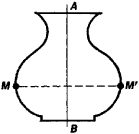Figure 1. Plane figure symmetric with respect to the line AB; reflection in AB carries the point M to M″

A reflection is an example of an orthogonal transformation that, in contrast to a direct motion, changes orientation. Any orthogonal transformation can be carried out by successively performing a finite number of reflections; this fact plays an important role in the investigation of the symmetry of geometric figures.

(2) Symmetry, in the broad sense, is the property of a geometric figure Φ that characterizes some regularity of the shape of Φ, or the invariance of Φ under motions. More precisely, Φ has symmetry, or is symmetric, if there exists a nontrivial orthogonal transformation that maps Φ onto itself. The set of all orthogonal transformations that map Φ onto itself is called the symmetry group of Φ; the transformations are themselves sometimes called symmetries.

Thus, if a plane figure is mapped onto itself under reflection, it is symmetric with respect to a line, which is the axis of symmetry (Figure 1). The symmetry group in this case consists of two elements. Suppose the plane figure Φ is such that rotations with respect to some point O through an angle of 360°/n, where n is an integer ≥ 2, map Φ onto itself; Φ is then said to have n-fold symmetry with respect to O, which is called the center of symmetry. Regular polygons (Figure 2) are examples of such figures; here, the symmetry group is a cyclic group of order n. A circle has infinite-fold symmetry, since it is mapped onto itself by rotation through any angle.

In addition to the symmetry produced by reflections, the simplest types of spatial symmetry include central symmetry, axial symmetry, and translational symmetry.

In the case of central symmetry (central inversion) or reflection in a point O, a figure Φ is mapped onto itself by successive reflections in three mutually perpendicular planes. In other words, O is the midpoint of the line segment joining symmetric points of Φ (Figure 3).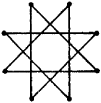Figure 2. Star-shaped regular polygon possessing eightfold symmetry with respect to its center

In the case of axial symmetry, or n-fold symmetry with respect to a line, a figure is mapped onto itself by rotation about some straight line, the axis of symmetry, through an angle of 360°/n. For example, the line AB is an axis of threefold symmetry, or threefold axis, of the cube, and the line CD is an axis of fourfold symmetry, or fourfold axis (Figure 3). In general, regular and semiregular polyhedrons are symmetric with respect to a number of lines. The location, number, and multiplicity of the axes of symmetry play an important role in crystallography.

A figure is said to have rotatory reflection symmetry if it can be mapped onto itself by a combination of a rotation through an angle of 360°/2k about a line AB and a reflection in a plane perpendicular to that line. A B is a 2k-fold rotoflection axis and a k-fold axis of symmetry (Figure 4). A twofold rotatory reflection is equivalent to a central inversion.

In the case of translational symmetry, the figure is mapped onto itself by translation along some line, the axis of translation. For example, a figure with a single translation axis has an infinite set of planes of symmetry, since any translation can be carried out by two successive reflections in planes perpendicular to the axis of translation (Figure 5). Figures that have several axes of translation play an important role in the investigation of crystal lattices.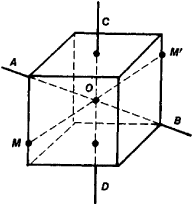Figure 3. Cube: (AB) a threefold axis of symmetry, (CD) a fourfold axis, (O) center of symmetry; the points M and M’ are symmetric with respect to AB, CD, and O

In art, symmetry is a widely used device for making a composition harmonious. Works of decorative applied art exhibit symmetry, as do works of architecture. Symmetry is an indispensable quality of a structure as a whole or at least of its parts and details—for example, of its plan, facade, columns, and capitals. Symmetry is also the basic device for making borders and ornament, which are plane figures that have, respectively, one or several translational symmetries combined with reflections.

Reflections and rotations can be used to generate all symmetries of figures. Combinations of symmetries generated by reflections, rotations, and translations are the subject of investigation in various fields of natural science. For example, a twist, which involves rotation through some angle about an axis followed by a translation along the axis, has a botanical application in phyllotaxy (literally, “leaf arrangement”). The symmetry of a molecular configuration affects the physical and chemical characteristics of the molecules and is of importance in the theoretical analysis of the structure of compounds, the properties of compounds, and the behavior of compounds in various reactions. The role of the geometric symmetry of crystals and lattices in the physical sciences has already been mentioned; in addition, concepts of symmetry in a more general sense are of great importance. Thus, the symmetry of space-time, as expressed in its homogeneity and isotropy (seeRELATIVITY, THEORY OF), makes possible the establishment of the conservation laws. Generalized symmetry plays an important role in the formation of atomic spectra and in the classification of elementary particles.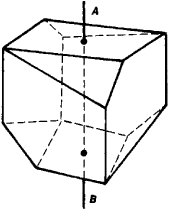Figure 4. Polyhedron possessing rotatory reflection symmetry: (AB) fourfold rotoflection axis

(3) Symmetry, in the general sense, means the invariance of the structure of a mathematical or physical object with respect to transformations of the object. For example, the symmetry of the laws of the theory of relativity is determined by their invariance with respect to the Lorentz transformations. The determination of the set of transformations that leave unchanged all structural relations of an object—that is, the determination of the group G of the object’s automorphisms—has become a guiding principle of contemporary mathematics and physics. This principle permits the internal structure of the object as a whole and of its parts to be studied more deeply.

Since such an object can be represented by the elements of some space P that possesses a corresponding characteristic structure, transformations of the object induce transformations of P. Thus, the representation of a group G in a group of transformations of P (or simply in P) is obtained, and the investigation of the symmetry of the object reduces to an investigation of the action of G on P and to a search for the invariants of this action. In like manner, the symmetry of the physical laws that control an object under investigation and that usually are described by the equations satisfied by the elements of P is determined by the action of G on such equations.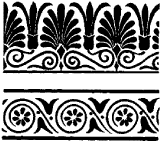Figure 5. Figures possessing translational symmetry; the upper figure also has an infinite set of vertical twofold axes of symmetry, that is, reflection planes

For example, if some equation is linear in a linear space P and remains invariant under the transformations of some group G, then to each element g of G there corresponds a linear transformation Tg in the linear space R of the solutions of the equation. The correspondence gTg is known as a linear representation of G. Knowledge of all such representations of G permits the establishment of various properties of the solutions and in many cases (on the basis of symmetry considerations) is of assistance in finding the solutions themselves. This fact, in particular, explains the need of mathematics and physics for a well developed theory of linear representations of groups.

REFERENCES

Shubnikov, A. V. Simmetriia (Zakony simmetrii i ikh primenenie v nauke, tekhnike i prikladnom iskusstve). Moscow-Leningrad, 1940.
Coxeter, H. S. M. Vvedenie v geometriiu. Moscow, 1966. (Translated from English.)
Weyl, H. Simmetriia. Moscow, 1968. (Translated from English.)
Wigner, E. Etiudy o simmetrii. Moscow, 1971. (Translated from English.) M. I. Voitsekhovskii

Symmetry

in mathematics and logic, the property of a binary (two-place, two-term) relation that indicates that the relation holds for any pair of objects regardless of the order in which the objects occur in the pair. In other words, a relation R is said to be symmetric if, for any objects x and y in its domain of definition, xRy implies yRx. Examples of symmetric relations include relations of the equality type—identity, equivalence, and similarity—and weak forms of such relations—for example, resemblance and proximity. These weak forms are known as tolerance relations. It follows from the definition of “symmetry” given above that the complements of the relations above are also symmetric; an example is the inequality relation.

The relation R is said to be antisymmetric if ⌉yRx (the negation of yRx) follows from xRy when xy—that is, if x = y invariably follows from xRy and yRx. Examples of antisymmetric relations are the relations of order (with respect to magnitude or some other ordering criterion) between numbers or other objects and the relation of inclusion between sets.

With respect to logical and logico-mathematical operations, the property of symmetry is called commutativity, or permuta-bility. For example, the results of the addition and multiplication of numbers, the union and intersection of sets, and the disjunction and conjunction of propositions (seeALGEBRA OF LOGIC) are independent of the order of the terms, factors, and so on. The concepts of symmetry and commutativity naturally extend to the case of an arbitrary number of objects.

Symmetry

in physics. In many cases, the laws that establish relations between quantities characterizing a physical system or that determine the variation of such quantities with time do not change under certain operations, or transformations, to which the system may be subjected. When this situation occurs, the laws are said to be symmetric, or invariant, under the given transformations. In mathematical terms, the symmetry transformations make up a group.

A description follows of the most general transformations under which physical laws have been observed to be symmetric.

Continuous transformations, TRANSLATION OF A SYSTEM AS A WHOLE IN SPACE. Two meanings can be given to the translation, or displacement, of a system in space, as well as to the other space-time transformations listed below. First, a translation can be understood as an active transformation—that is, as the actual translation of a physical system with respect to a chosen frame of reference. Second, a translation can be understood as a passive transformation, wherein the frame of reference undergoes translation. The symmetry of physical laws under displacements in space means that all points in space are equivalent. In other words, space is homogeneous—it possesses no distinctive points.

ROTATION OF A SYSTEM AS A WHOLE IN SPACE. The symmetry of physical laws under rotation means that all directions in space are equivalent—that is, space is isotropic.

TRANSLATION IN TIME. Symmetry under a translation in time, or a change in the origin of the time coordinate, means that physical laws do not change with time.

LORENTZ TRANSFORMATIONS. The Lorentz transformations involve a change from a given frame of reference to a frame moving with constant velocity (constant in direction and magnitude) relative to the given frame. Symmetry under these transformations means, in particular, that all inertial frames of reference are equivalent (seeRELATIVITY, THEORY OF).

GAUGE TRANSFORMATIONS. The laws that describe the interaction of particles possessing some charge (electric charge, bary-onic charge, leptonic charge, or hypercharge) are invariant under gauge transformations of the first kind. In such a transformation, the wave functions of all particles are multiplied simultaneously by an arbitrary phase factor: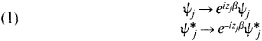Here, ψj is the wave function of particle j, ψ*j is its complex conjugate function, β is an arbitrary numerical factor, and zj is the charge corresponding to the particle—zj is expressed in units of elementary charge, for example, the elmentary electric charge e.

In addition, electromagnetic interactions are symmetric under gauge transformations of the second kind (gradient transformations) for electromagnetic field potentials (A, Φ):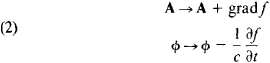Here, f (x, y, z, t) is an arbitrary function of the coordinates x, y and z and the time t, and c is the speed of light. In order for transformations (1) and (2) to be satisfied simultaneously in the case of electromagnetic fields, the gauge transformations of the first kind must be generalized. Specifically, the interaction laws must be invariant under transformations (1) with a quantity β that is an arbitrary function of the coordinates and time: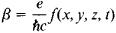where ħ is Planck’s constant. The relation between gauge transformations of the first and second kinds for electromagnetic interactions results from the dual role of the electric charge. On the one hand, the electric charge is a conserved quantity; on the other hand, it is the coupling constant, which characterizes the interaction of the electromagnetic field with charged particles.

Transformations (1) correspond to the laws of conservation of various charges (see below) and some internal interaction symmetries. If the charges are not only conserved quantities but also sources of fields (like the electric charge), then the fields corresponding to the charges should be gauge fields, in much the same way as electromagnetic fields are gauge fields. Transformations (1) here are generalized to the case where the quantities β are arbitrary functions of the coordinates and time and may even be operators transforming the states of internal symmetry. In the theory of interacting fields, such an approach leads to various gauge theories of the strong and weak interactions, in particular, the Yang-Mills theory.

ISOTOPIC-SPIN INVARIANCE OF STRONG INTERACTIONS. Strong interactions are symmetric under rotations in what is known as isotopic space. One of the manifestations of this isotopic-spin, or isospin, symmetry is the charge independence of the nuclear forces, which consists in the equivalence of strong neutron-neutron, proton-proton, and neutron-proton interactions when the particles are in the same states. Isotopic-spin invariance is an approximate symmetry and is violated by electromagnetic interactions. It is part of the broader approximate symmetry of strong interactions called SU(3) symmetry (see).

Discrete transformations. The types of symmetry mentioned above are characterized by parameters that can vary continuously in some range of values. For example, translation in space is characterized by the three parameters of displacement along the coordinate axes, and rotation is characterized by the three angles of rotation about these axes. In addition to continuous symmetries, discrete symmetries are of great importance in physics. A description follows of the principal discrete symmetries.

Space inversion. Processes resulting from the strong and electromagnetic interactions are symmetric under space inversion (P). Such processes are described identically in the two different Cartesian coordinate systems that can be obtained from each other by reversing the directions of the coordinate axes. Under space inversion a right-handed coordinate system changes to a left-handed coordinate system. Since space inversion can be understood as reflections in the origin, symmetry with respect to space inversion is sometimes called reflection symmetry. Invariance under space inversion means that if some process due to a strong or electromagnetic interaction occurs in nature, then the process that is the mirror image of this process occurs with the same probability. The physical quantities characterizing the two processes are related in a definite manner. For example, particle velocities and electric field strengths reverse direction under space inversion, but the directions of the magnetic field strength and angular momentum remain unchanged.

Certain phenomena occurring in isomeric substances would appear to violate reflection symmetry. In optical isomerism, for example, the plane of polarization of light is rotated to the right or to the left. In actuality, however, reflection symmetry is not violated in such phenomena: for any levorotatory substance, for example, there exists a corresponding dextrorotatory substance. The dextrorotatory substance has the same chemical composition as the levorotatory substance, and the molecules of the dextrorotatory substance are mirror images of the molecules of the levorotatory substance.

Space-inversion symmetry is violated by weak-interaction processes.

CHARGE CONJUGATION. In charge conjugation (C), particles are replaced by their antiparticles. Processes due to strong and electromagnetic interactions are invariant under charge conjugation. Weak-interaction processes, however, violate charge conjugation symmetry. Under charge conjugation, the charges of particles and the electric and magnetic field strengths are replaced by their opposite values.

COMBINED INVERSION. The successive performance of space inversion and charge conjugation is known as combined inversion (CP). Since the strong and electromagnetic interactions are symmetric with respect to space inversion and charge conjugation, they are also symmetric with respect to combined inversion. Although weak interactions are not invariant under space inversion and charge conjugation separately, they are invariant under combined inversion. The symmetry of weak-interaction processes with respect to combined inversion may indicate that the violation of space inversion symmetry by such interactions is associated with the structure of elementary particles and that antiparticles are in structure the mirror image of the corresponding particles. In this sense, weak interactions between particles and the corresponding weak interactions between the antiparticles exhibit the same relation as phenomena in optical isomers.

The decay of the long-lived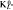-mesons into two π-mesons and the charge asymmetry in the decays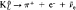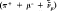and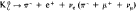(seeKAONS) indicate the existence of forces that are not invariant under combined inversion. It has not yet been established whether these forces are small additions to the known fundamental interactions (the strong, electromagnetic, and weak interactions) or whether they have a special nature. Nor can the possibility be excluded that this violation of combined inversion symmetry is due to special geometric properties of space-time over small intervals.

TIME REVERSAL. The time reversal operation (T) changes the sign of time. All elementary processes occurring as a result of the strong, electromagnetic, and weak interactions (with the exception of decays ofmesons) are invariant under time reversal.

CPT. The product of the three operations charge conjugation C, space inversion P, and time reversal T is known as CPT. CPT symmetry follows from the general principles of quantum field theory and is connected primarily with invariance under the Lorentz transformations and with the locality of the interaction—that is, with the interaction of the fields at a single point. This symmetry should hold even if the interactions are not invariant under charge conjugation, space inversion, and time reversal taken separately.

A consequence of CPT invariance is crossing symmetry in the description of processes involving particles and antiparticles. Thus, a single analytic function describes the following three reactions: the elastic scattering of some particle a by some particle b, a + b → a + b; the elastic scattering of the antiparticle by the particle b, ā + b → ā + b; and the annihilation of the particle a and its antiparticle ā with the formation of the pair of particles b and, a + ā → b + b. This analytic function is dependent on the square of the total energy of the system and the square of the transferred momentum. In different ranges of these variables the function gives the amplitude of each of the above processes.

INTERCHANGE OF IDENTICAL PARTICLES. The wave function of a system containing identical particles is symmetric with respect to the interchange of any pair of particles (that is, the interchange of the particles’ coordinates and spins) when the particles have an integral—in particular, zero—spin. The wave function is said to be antisymmetric when the particles have a half-integral spin (seeQUANTUM MECHANICS).

Symmetry and conservation laws. According to Noether’s theorem, to every symmetry transformation characterized by one continuously varying parameter there corresponds a quantity that is conserved (does not vary with time) in the system possessing this symmetry. The laws of conservation of momentum, angular momentum, and energy follow from the invariance of physical laws under a displacement of a closed system in space, a rotation of the system as a whole, and a change in the origin of the time coordinate, respectively. The laws of conservation of charges, such as electric and baryonic charges, follow from invariance under gauge transformations of the first kind. The conservation of isotopic spin in strong interactions follows from isotopic-spin invariance.

The discrete symmetries do not lead to any conservation laws in classical mechanics. The situation is different in quantum mechanics, where the state of a system is described by a wave function, and in the case of wave fields, such as the electromagnetic field, where the superposition principle is valid. In these cases, some specific quantities obey conservation laws that have no analogues in classical mechanics. The existence of such quantities can be demonstrated through the example of space parity, whose conservation follows from invariance under space inversion. Suppose ψ1, is a wave function describing some state of a system and ψ2 is the system’s wave function obtained as a result of space inversion: ψ2 = P̂Ψ1 where is the space inversion operator. Then, if space inversion symmetry holds, ψ2 is one of the possible states of the system. It follows from the superposition principle that the superpositions of ψ1, and ψ2 are possible states of the system. These superpositions are the symmetric combination ψs = ψ1 + ψ2, and the antisymmetric combination ψa = ψ1 – ψ2. The state ψs does not change under space inversion, since Ψs = P̂Ψ1 + P̂Ψ1 + Ψ2 = ψ2 + Ψ1 = ψs. The state ψa, however, changes sign under this operation, since Ψa = P̂ψ1 + P̂ψ2 = Ψ2 - Ψ1 = ψa. In the case where the sign does not change, the space parity of the system is + 1 and is said to be even; in the case where the sign changes, the parity is - 1 and is said to be odd. If the wave function of a system is given by means of quantities that are invariant under space inversion (such as angular momentum and energy), then the parity of the system also has a well-defined value. The system in this case is in a state with either even or odd parity, and transitions from one state to the other under the action of inversion-invariant forces are forbidden.

Similarly, charge parity and combined parity follow from invariance under charge conjugation and combined inversion, respectively. These quantities, however, can be used to characterize only absolutely neutral particles or systems—that is, particles or systems where all charges have the value zero. Since under charge conjugation a system with a nonzero charge becomes a system with a charge of opposite sign, a superposition of these two states cannot be formed without violating the law of conservation of charge. The concept of isotopic parity, or C parity, can be introduced to characterize a system of strongly interacting particles, or hadrons, that have zero baryonic charge, zero strangeness or hypercharge, and nonzero electric charge. This concept arises from charge conjugation and the isotopic-spin invariance of strong interactions (isotopic-spin invariance can be interpreted as symmetry with respect to rotation in isotopic space). The pion is an example of such a system.

Symmetry of quantum-mechanical systems and stationary states; degeneracy. The conservation of quantities corresponding to different symmetries of a quantum-mechanical system is a consequence of the principle that the operators corresponding to the symmetries commute with the Hamiltonian of the system if the Hamiltonian is not explicitly time-dependent (seeQUANTUM MECHANICS and COMMUTATION RELATIONS). Accordingly, these quantities can be measured simultaneously with the energy of the system—that is, they can assume well defined values for a given energy value. These quantities can therefore be used to form a complete set of quantities defining the state of the system. Thus, the system’s stationary states—that is, states with a definite energy—are determined by quantities corresponding to symmetries of the system.

When a quantum-mechanical system has symmetry, the different states of motion of the system obtained from each other by symmetry operations have identical values for the physical quantities that are invariant under the operations. Thus, the symmetry of a system generally leads to degeneracy. For example, to a certain value of the energy of the system there may correspond several different states that are carried into each other by the symmetry operations. In mathematical terms, these states are the basis of an irreducible representation of the symmetry group of the system (seeGROUP). This fact accounts for the fruitfulness of the application of the methods of group theory in quantum mechanics.

In addition to the degeneracy of energy levels that is connected with an explicit symmetry of a system (for example, symmetry with respect to rotations of the system as a whole), in many problems an additional degeneracy exists that is associated with a latent symmetry of an interaction. Such latent symmetries exist, for example, for the Coulomb interaction and for an isotropic oscillator.

If a system possessing some symmetry is located in a field of forces that violate this symmetry but are sufficiently weak to be considered a small perturbation, the degenerate energy levels of the original system split. Under the action of an “asymmetric” perturbation, the various states that have the same energy owing to the system’s symmetry acquire different energy displacements. When the perturbing field has some symmetry forming a part of the symmetry of the original system, the degeneracy of the energy levels is not completely removed: some of the levels remain degenerate, in accordance with the symmetry of the interaction that includes the perturbing field.

The presence of energy-degenerate states in a system indicates the existence of an interaction symmetry. In principle, this symmetry can be found when it is not known in advance—a circumstance that plays a very important role in, for example, elementary particle physics. On the basis of the existence of isotopic-spin multiplets (that is, groups of particles having different electric charges, similar masses, and identical other characteristics), it was possible to establish the isotopic-spin invariance of strong interactions. The possibility of uniting particles with identical properties in larger groups led to the discovery of the SU(3) symmetry of the strong interaction and to the finding of interactions that violate this symmetry (seeSTRONG INTERACTIONS). Grounds exist for believing that the strong interaction has an even larger symmetry group.

The concept of the dynamical symmetry of a system is extremely fruitful. This concept arises in the consideration of transformations that include transitions between states of a system with different energies. The spectrum of the stationary states of the system is an irreducible representation of the group of dynamical symmetries. The concept of dynamical symmetry can also be extended to cases where the Hamiltonian of the system is explicitly time-dependent. Here, all states of a quantum-mechanical system that are not stationary states (that is, that do not have a definite energy) are united in one irreducible representation of the group of dynamical symmetries.

REFERENCCE

Wigner, E. Etiudy o simmetrii. Moscow, 1971. (Translated from English.)

C. C. GERSHTEIN

symmetry

[′sim·ə‚trē]
(biology)
The disposition of organs and other constituent parts of the body of living organisms with respect to imaginary axes.
(mathematics)
A geometric object G has this property relative to some configuration S of its points if S determines two pieces of G which can be reflected onto each other through S.
A rigid motion of a geometric figure that maps the figure onto itself.
(physics)
McGraw-Hill Dictionary of Scientific & Technical Terms, 6E, Copyright © 2003 by The McGraw-Hill Companies, Inc.

symmetry

1. Maths an exact correspondence in position or form about a given point, line, or plane
2. Physics the independence of a property with respect to direction; isotropy
Collins Discovery Encyclopedia, 1st edition © HarperCollins Publishers 2005
References in periodicals archive ?
In  inviscid, compressible, laminar, axisymmetric steady swirling flow is numerically investigated.
Since the AFM cantilever tip is a pyramid, the projection area A of the tip-sample contact surface is not circular, that is, not axisymmetric. However, numerical analysis [29, 30] indicates that Figure 4(a) could be approximated by the contact between a conic indenter and substrate material as illustrated in Figure 4(b) with a negligible error of 0.012, as long as the conic gives the same projected area-to-depth ratio A/h as that of pyramid.
Whereas for axisymmetric class of BHs including AdS spacetime there has been no chance to formulate any possible combinations of thermodynamic volume product to be mass-independent, it should be noted that, for a KN-AdS BH, there may be a possibility of formulating the area (or entropy) product relations to be mass-independent.
Then axisymmetric Hall-MHD equations are reduced to the following:
Testing the correctness of integral equations for calculating the distribution of fictitious magnetic charges on the axisymmetric boundaries of a piecewise homogeneous magnetized medium and the algorithms of their numerical solutions can be carried out using analytical solutions of problems of analyzing the action of homogeneous electrostatic field on a piecewise homogeneous dielectric medium with a central symmetry of boundaries - single-layer and multilayer spherical shells.
The initial axisymmetric vortex is in hydrostatic and gradient wind balance.
The numerical simulations of the flow inside the chamber have been carried out in a 2D (2-dimensional) axisymmetric computational domain.
Our objective of this article is to prepare and utilize the proposed methods to obtain an analytical solution to the fractional form of an unsteady axisymmetric flow of nonconducting, Newtonian fluid squeezed between two circular plates passing through porous medium with slip and no-slip boundary conditions.
A numerical analysis of axisymmetric transverse-free vibration of the circular membrane with nonhomogeneous changing density was analyzed by Li [14, 15], and the first-order natural frequencies were given.

Site: Follow: Share:
Open / Close